# PSAT Math : Integers

## Example Questions

1 2 11 12 13 14 15 16 17 19 Next →

### Example Question #1 : How To Find The Next Term In An Arithmetic Sequence

Find the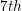term in the sequence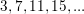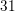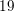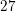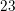Explanation:

Notice that in the sequenceeach term increases by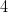.

It is always good strategy when attempting to find a pattern in a sequence to examine the difference between each term.

We continue the pattern to find:

The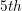term isThe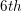term isTheterm isIt is useful to note that the sequence is defined by,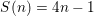where n is the number of any one term.

We can solve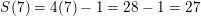to find theterm.

1 2 11 12 13 14 15 16 17 19 Next →

### All PSAT Math Resources